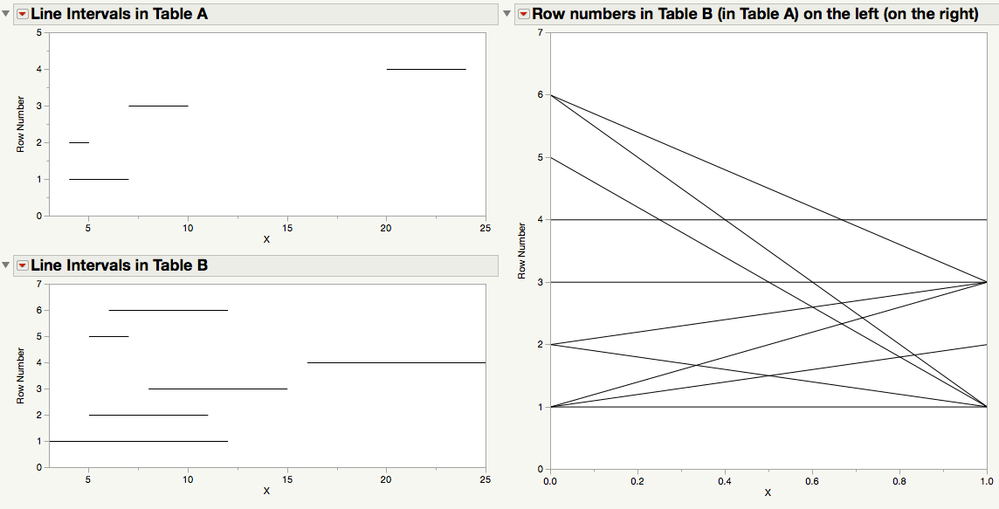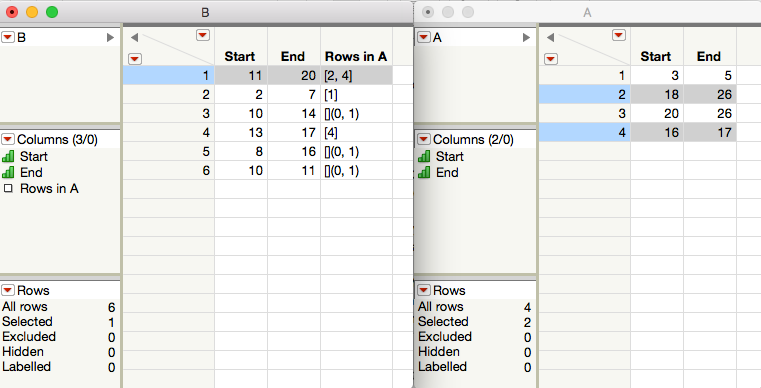Our World Statistics Day conversations have been a great reminder of how much statistics can inform our lives. Do you have an example of how statistics has made a difference in your life? Share your story with the Community!
Choose Language Hide Translation Bar
Highlighted

## Overlapping Times

Say I have two tables. Table A and Table B. Both table A and B have event start and end times for events. I want to generate a new table that contains only events from table B that occur during the same time frame as events on table A. Is there an easy way to do this with JSL?

2 ACCEPTED SOLUTIONS

Accepted Solutions
Highlighted

## Re: Overlapping Times

This might be better. It uses integer time values, but that's OK.``````NamesDefaultToHere(1);

dtA = NewTable("A",
NewColumn("Start", Numeric, Ordinal, Formula(RandomInteger(1, 20))),
NewColumn("End", Numeric, Ordinal, Formula(:Start + RandomInteger(1, 10)))
);
dtA << runFormulas;
dtA:Start << deleteFormula;
dtA:End << deleteFormula;

dtB = NewTable("B",
NewColumn("Start", Numeric, Ordinal, Formula(RandomInteger(1, 20))),
NewColumn("End", Numeric, Ordinal, Formula(:Start + RandomInteger(1, 10)))
);
dtB << runFormulas;
dtB:Start << deleteFormula;
dtB:End << deleteFormula;

// Get values into matrices
matA = dtA << getAsMatrix;
matB = dtB << getAsMatrix;

// Loop over the events in 'B' and compare each with all those in 'A' at the same time
matchesBtoA = AssociativeArray();
for(e=1, e<=NRow(matB), e++,
startB = matB[e,1];
endB = matB[e,2];
matchesInA = Loc((matA[0,1] < endB) & (startB < matA[0,2]));
matchesBtoA << InsertItem(e, matchesInA);
);

// Graphs
maxX = Maximum(Maximum(matA[0,2]), Maximum(matB[0,2]));
minX = Minimum(Minimum(matA[0,1]), Minimum(matB[0,1]));
NewWindow("Overlapping Intervals", << onClose(Close(dtA, NoSave); Close(dtB, NoSave)),
LineUpBox(NCol(2),
VListBox(
GraphBox(Title("Line Intervals in Table A"),
XScale(minX, maxX), YScale(0, NRow(matA)+1), YName("Row Number"), FrameSize(500, 210),
for(ii = 1, ii<=NRow(matA), ii++,
Line({matA[ii,1], ii}, {matA[ii,2], ii});
);
),
GraphBox(Title("Line Intervals in Table B"),
XScale(minX, maxX), YScale(0, NRow(matB)+1), YName("Row Number"), FrameSize(500, 210),
for(ii = 1, ii<=NRow(matB), ii++,
Line({matB[ii,1], ii}, {matB[ii,2], ii});
);
)
),
GraphBox(Title("Row numbers in Table B (in Table A) on the left (on the right)"),
XScale(0, 1), YScale(0, Maximum(NRow(matA), NRow(matB))+1), YName("Row Number"), FrameSize(500, 500),
for(ii = 1, ii<=NRow(matB), ii++,
lines = matchesBtoA << getValue(ii);
for(jj=1, jj<=NRow(lines), jj++,
Line({0, ii}, {1, lines[jj]});
);
);
)
)
);
```. ```

Highlighted

## Re: Overlapping Times

You could use a row state handler (see below). In so far as the matches are persisted in 'Table B', one would have to be concerned about updating these when the data in either 'Table B' or 'Table A' changes. Also, even though the linking is bidirectional in concept, if you try to add a corresponding row state handler to 'Table A', unsurprisingly perhaps, they interfere with each other.

Using this code, selections in 'Table B' will propagate to 'Table A':``````NamesDefaultToHere(1);

dtA = NewTable("A",
NewColumn("Start", Numeric, Ordinal, Formula(RandomInteger(1, 20))),
NewColumn("End", Numeric, Ordinal, Formula(:Start + RandomInteger(1, 10)))
);
dtA << runFormulas;
dtA:Start << deleteFormula;
dtA:End << deleteFormula;

dtB = NewTable("B",
NewColumn("Start", Numeric, Ordinal, Formula(RandomInteger(1, 20))),
NewColumn("End", Numeric, Ordinal, Formula(:Start + RandomInteger(1, 10)))
);
dtB << runFormulas;
dtB:Start << deleteFormula;
dtB:End << deleteFormula;

// Get values into matrices
matA = dtA << getAsMatrix;
matB = dtB << getAsMatrix;

// Loop over the events in 'B' and compare each with all those in 'A' at the same time
matchesBtoA = AssociativeArray();
for(e=1, e<=NRow(matB), e++,
startB = matB[e,1];
endB = matB[e,2];
matchesInA = Loc((matA[0,1] < endB) & (startB < matA[0,2]));
matchesBtoA << InsertItem(e, matchesInA);
);

// Persist the matches in 'B', which becomes the 'master table'
dtB << New Column( "Rows in A", Expression);
for (r=1, r<=NRow(matB), r++,
Column(dtB, "Rows in A")[r] = matchesBtoA << getValue(r);
);

// This row state handler detects row selections in 'B' and selects rows in 'A'
fB =
function({sourceRows}, {Default Local},
selectedSourceRows = dtB << getSelectedRows;
rows2select = [];
for(r=1, r<=NRow(selectedSourceRows), r++,
rows2select = VConcat(rows2select, Column(dtB, "Rows in A")[selectedSourceRows[r]]);
);
dtA << clearSelect;
dtA << selectRows(rows2select)
);

// Assign row state handler to 'B'
obB = dtB << MakeRowStateHandler(fB);
``````
10 REPLIES 10
Highlighted

## Re: Overlapping Times

Edit: I probably solved a different problem than you describe.  In your example, does each row have a (start,end) and you want to intersect rows in table B with all rows in table A?  In my example there is only one collective (start,end) for the table..

Original response:

Here's one way to create the new table:

``````seriesP = Open("\$SAMPLE_DATA/Time Series/SeriesP.jmp");
seriesP_years = seriesP:Year << Get Values;
seriesP_min = Min(seriesP_years);
seriesP_max = Max(seriesP_years);

seriesR = Open("\$SAMPLE_DATA/Time Series/SeriesR.jmp");
seriesR << Select Where(:Year >= seriesP_min & :Year <= seriesP_max);
seriesRsubset = seriesR << Subset("Selected Rows");``````

Highlighted

## Re: Overlapping Times

Yes, both tables have a start and end time for each event. So I need a solution that finds events in table B which are inside or overlapped by events in table A.

Highlighted

## Re: Overlapping Times

FYI, here is how I am doing it now.

``````OverlappingEvents = Function( {PrimaryTableName, PrimaryTableStartTime, PrimaryTableEndTime, SecondaryTableName, SecondaryTableStartTime, SecondaryTableEndTime}, {},
SecondaryTableName << Clear Selection;
For(i = 1, i <= nrows(PrimaryTableName), i++,
::PrimaryEndTime = Column( PrimaryTableName, PrimaryTableEndTime )[i];
::PrimaryStartTime = Column( PrimaryTableName, PrimaryTableStartTime )[i];
SecondaryTableName << select where( ::PrimaryStartTime <= as column(SecondaryTableStartTime) & as column(SecondaryTableEndTime) <= ::PrimaryEndTime | ::PrimaryStartTime <= as column(SecondaryTableEndTime) <= ::PrimaryEndTime | ::PrimaryStartTime <= as column(SecondaryTableStartTime) <= ::PrimaryEndTime, Current selection("Extend") ); //select all events in secondary table that overlap or are within events on primary table
);
);``````

Unfortunately, the function takes a very long time when working with large datatables. Is there a better way?

Highlighted

## Re: Overlapping Times

This might be better. It uses integer time values, but that's OK.``````NamesDefaultToHere(1);

dtA = NewTable("A",
NewColumn("Start", Numeric, Ordinal, Formula(RandomInteger(1, 20))),
NewColumn("End", Numeric, Ordinal, Formula(:Start + RandomInteger(1, 10)))
);
dtA << runFormulas;
dtA:Start << deleteFormula;
dtA:End << deleteFormula;

dtB = NewTable("B",
NewColumn("Start", Numeric, Ordinal, Formula(RandomInteger(1, 20))),
NewColumn("End", Numeric, Ordinal, Formula(:Start + RandomInteger(1, 10)))
);
dtB << runFormulas;
dtB:Start << deleteFormula;
dtB:End << deleteFormula;

// Get values into matrices
matA = dtA << getAsMatrix;
matB = dtB << getAsMatrix;

// Loop over the events in 'B' and compare each with all those in 'A' at the same time
matchesBtoA = AssociativeArray();
for(e=1, e<=NRow(matB), e++,
startB = matB[e,1];
endB = matB[e,2];
matchesInA = Loc((matA[0,1] < endB) & (startB < matA[0,2]));
matchesBtoA << InsertItem(e, matchesInA);
);

// Graphs
maxX = Maximum(Maximum(matA[0,2]), Maximum(matB[0,2]));
minX = Minimum(Minimum(matA[0,1]), Minimum(matB[0,1]));
NewWindow("Overlapping Intervals", << onClose(Close(dtA, NoSave); Close(dtB, NoSave)),
LineUpBox(NCol(2),
VListBox(
GraphBox(Title("Line Intervals in Table A"),
XScale(minX, maxX), YScale(0, NRow(matA)+1), YName("Row Number"), FrameSize(500, 210),
for(ii = 1, ii<=NRow(matA), ii++,
Line({matA[ii,1], ii}, {matA[ii,2], ii});
);
),
GraphBox(Title("Line Intervals in Table B"),
XScale(minX, maxX), YScale(0, NRow(matB)+1), YName("Row Number"), FrameSize(500, 210),
for(ii = 1, ii<=NRow(matB), ii++,
Line({matB[ii,1], ii}, {matB[ii,2], ii});
);
)
),
GraphBox(Title("Row numbers in Table B (in Table A) on the left (on the right)"),
XScale(0, 1), YScale(0, Maximum(NRow(matA), NRow(matB))+1), YName("Row Number"), FrameSize(500, 500),
for(ii = 1, ii<=NRow(matB), ii++,
lines = matchesBtoA << getValue(ii);
for(jj=1, jj<=NRow(lines), jj++,
Line({0, ii}, {1, lines[jj]});
);
);
)
)
);
```. ```

Highlighted

## Re: Overlapping Times

I think the overlap conditions in the solutions are slightly different - The matrix condition does not count overlap if the two intervals only touch (< vs. <=), and the Select Where() does not count a B interval that wholly contains an A interval. Either way I think the matrix approach will be faster, but both will bog down for very large tables.  To do much better would require some sorting and data structures like the Interval Tree.  Doing that from JSL might be more costly than the brute force methods..

Highlighted

## Re: Overlapping Times

Thanks for the interesting approach. I am trying to wrap my head around the following line:

``matchesInA = Loc((matA[0,1] < endB) & (startB < matA[0,2]));``

What is it doing, and how does it work? I appreciate your help!

Highlighted

## Re: Overlapping Times

TBH, it took me a few attempts (and a pen and paper) to get the logic right. Just draw two line segments in all the various configurations (including when A is wholy contained in B, or vice versa). Actually, that's why I added the graphs to be sure I'd got it right!

Using matrix operations allows you to vectorise things, which is generally faster). In terms of the syntax, matA[0,1] (matA[0,2]) contains all the start (end) points for the line segments denoted as 'A'. The 'Loc()' function returns a column vector giving the row numbers in matA for which the logical condition is satisfied.

As Dan mentioned, you could use <= instaed of < depending on what you want the result to be.

Highlighted

## Re: Overlapping Times

Thank you! I have now made the associative array work, and it is MUCH faster than what I was doing before (ie. 2 minutes vs 2 hours).

Is there a way I could take the associative array and use it to automatically select the corresponding row numbers in Table B?

Highlighted

## Re: Overlapping Times

You could use a row state handler (see below). In so far as the matches are persisted in 'Table B', one would have to be concerned about updating these when the data in either 'Table B' or 'Table A' changes. Also, even though the linking is bidirectional in concept, if you try to add a corresponding row state handler to 'Table A', unsurprisingly perhaps, they interfere with each other.

Using this code, selections in 'Table B' will propagate to 'Table A':``````NamesDefaultToHere(1);

dtA = NewTable("A",
NewColumn("Start", Numeric, Ordinal, Formula(RandomInteger(1, 20))),
NewColumn("End", Numeric, Ordinal, Formula(:Start + RandomInteger(1, 10)))
);
dtA << runFormulas;
dtA:Start << deleteFormula;
dtA:End << deleteFormula;

dtB = NewTable("B",
NewColumn("Start", Numeric, Ordinal, Formula(RandomInteger(1, 20))),
NewColumn("End", Numeric, Ordinal, Formula(:Start + RandomInteger(1, 10)))
);
dtB << runFormulas;
dtB:Start << deleteFormula;
dtB:End << deleteFormula;

// Get values into matrices
matA = dtA << getAsMatrix;
matB = dtB << getAsMatrix;

// Loop over the events in 'B' and compare each with all those in 'A' at the same time
matchesBtoA = AssociativeArray();
for(e=1, e<=NRow(matB), e++,
startB = matB[e,1];
endB = matB[e,2];
matchesInA = Loc((matA[0,1] < endB) & (startB < matA[0,2]));
matchesBtoA << InsertItem(e, matchesInA);
);

// Persist the matches in 'B', which becomes the 'master table'
dtB << New Column( "Rows in A", Expression);
for (r=1, r<=NRow(matB), r++,
Column(dtB, "Rows in A")[r] = matchesBtoA << getValue(r);
);

// This row state handler detects row selections in 'B' and selects rows in 'A'
fB =
function({sourceRows}, {Default Local},
selectedSourceRows = dtB << getSelectedRows;
rows2select = [];
for(r=1, r<=NRow(selectedSourceRows), r++,
rows2select = VConcat(rows2select, Column(dtB, "Rows in A")[selectedSourceRows[r]]);
);
dtA << clearSelect;
dtA << selectRows(rows2select)
);

// Assign row state handler to 'B'
obB = dtB << MakeRowStateHandler(fB);
``````
Article Labels

There are no labels assigned to this post.